# Division

Division is sharing things equally between parts or groups.

For example, if there are 12 baby pandas and 4 wildlife sanctuaries want to share them before releasing them into the wild, we can split them up equally as follows: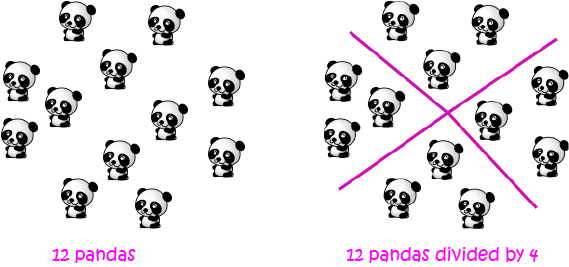So, each of the four sanctuaries will receive three baby pandas. We say that $12$ divided by $4$ is $3$.

## Symbols for DivisionWe use the symbols $\div$ and $/$ for division. So, we can write:

\begin{align*} 12 \div 4 &= 3 \text{ or}\\ 12/4 &= 3 \end{align*}.

Your work books will most often use the symbol $\div$.

## Division as Reverse Multiplication

Division and multiplication are opposites of each other. So, division provides a way to "undo" multiplication, and multiplication provides a way to "undo" division.

When we know a division fact, we can work out multiplication facts, and when we know multiplication facts, we can work out division facts.

For example, we know that $12 \div 4 = 3$. The related multiplication fact is $4 \times 3 = 12$.

Let's try using this multiplication to work out some related division facts: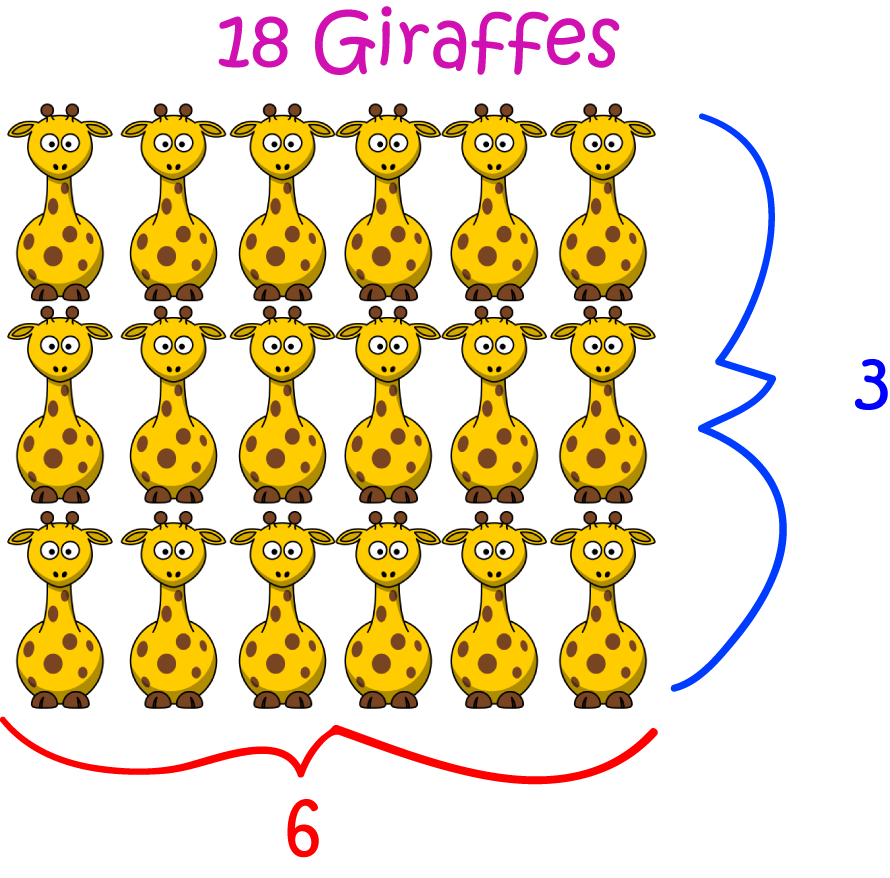The three rows of six giraffes make $18$ giraffes. So, 18 divided by $3$ is $6$.

There are six columns of three giraffes, making $18$ giraffes. So, $18$ divided by $6$ is $3$.

So, we have four related division and multiplication facts:

• $3 \times 6 = 18$
• $6 \times 3 = 18$
• $18 \div 3 = 6$
• $18 \div 6 = 3$

Let's try another one. In the picture below, there are six gummi bears arranged in rows.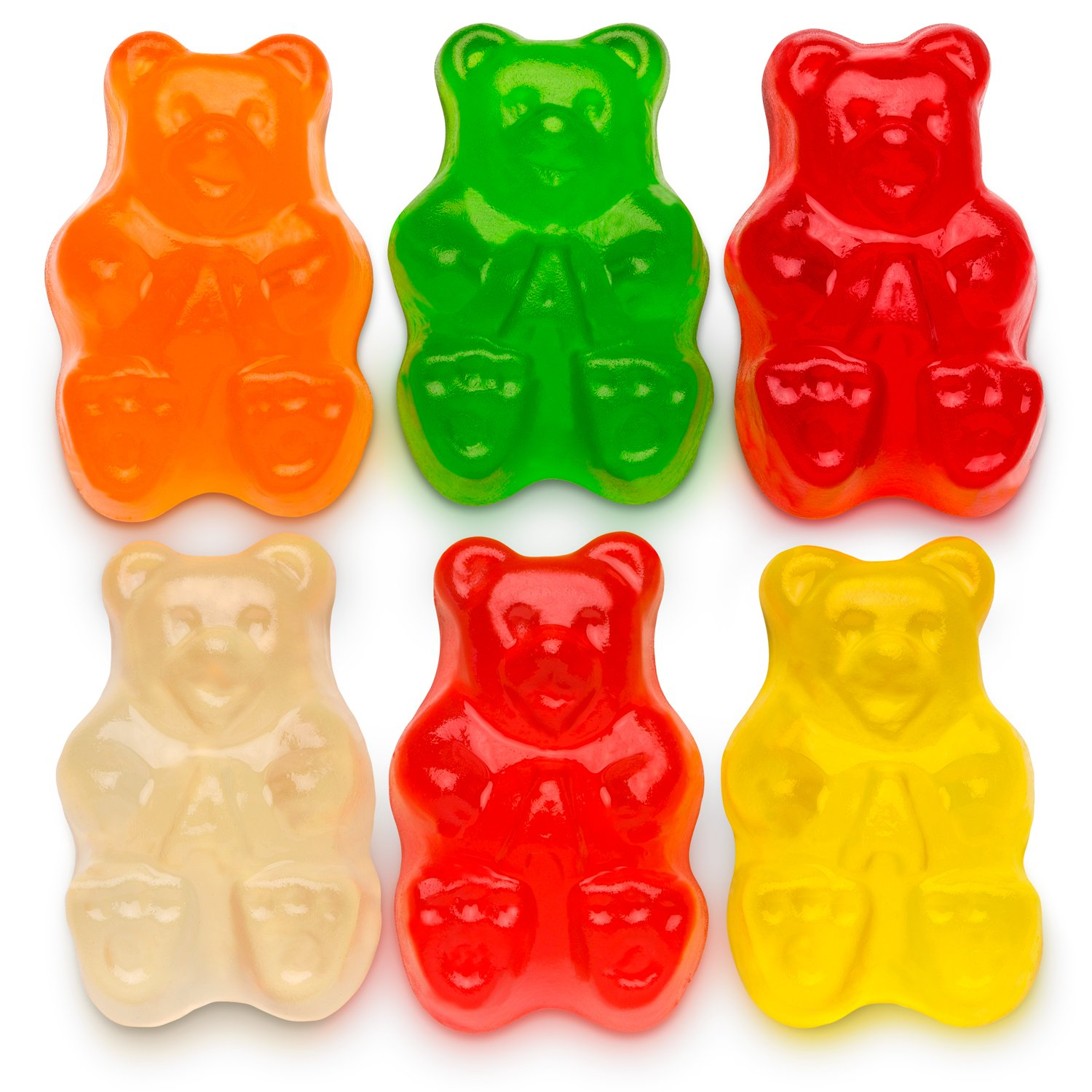The two rows of three gummi bears make six gummi bears. So, $6$ divided by $2$ is $3$.

The three columns of two gummi bears make six gummi bears. So, $6$ divided by $3$ is $2$.

So, we have four related division and multiplication facts:

• $3 \times 2 = 6$
• $2 \times 3 = 6$
• $6 \div 3 = 2$
• $6 \div 2 = 3$

You guessed it! If you want to be good at division, it's a really good idea to learn your times tables.

Let's see another example of how you can use your times tables to work out a division sum.

For example, what is $33 \div 3$?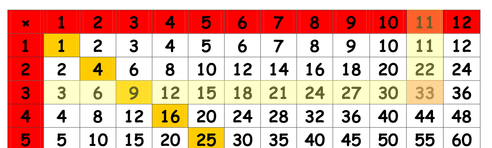If we look along the $3$s row of the times table grid, we can eventually find $33$.

It's in the column under the $11$, so $3 \times 11 = 33$. This tells us that $33$ divided by $3$ is $11$.

Our answer is: $33 \div 3 = 11$.

### Names for the parts of a division sum

Just like addition, subtraction and multiplication, the parts of a division sum have special names. Unfortunately, they aren't nice easy names like "Fred" and "Alice", so they're a bit difficult to remember. Here they are:

$\text{dividend} \div \text{divisor} = \text{quotient}$.
The most important thing to remember is that the quotient is the answer.

For example, in $6 \div 3 = 2$:

• $6$ is the dividend.
• $3$ is the divisor.
• $2$ is the quotient.

### What if things can't be shared evenly?

If you can't share things evenly, you'll end up with some of them left over. The stuff that's left over is called the remainder.

For example, Gus and Sam the snails have $5$ cabbages to share between them. But, you can't share $5$ cabbages evenly between $2$ snails.

Each snail gets $2$ cabbages,

and there is $1$ left over. There's a remainder of $1$.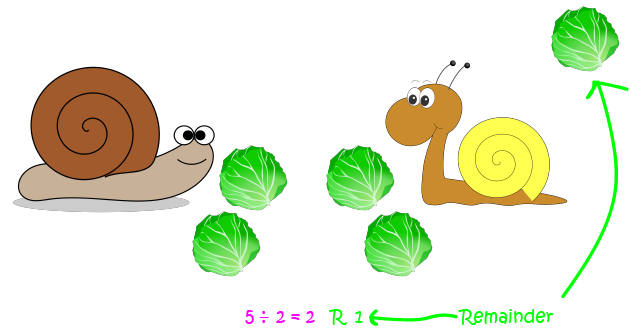### Description

This mini book covers the core of Math for Foundation, Grade 1 and Grade 2 mathematics including

1. Numbers
3. Subtraction
4. Division
5. Algebra
6. Geometry
7. Data
8. Estimation
9. Probability/Chance
10. Measurement
11. Time
12. Money
13. and much more

This material is provided free of cost for Parent looking for some tricks for their Prekinder, Kinder, Prep, Year 1 and Year 2 children

### Learning Objectives

These lessons are for kids aged 4-8 with the core objective to expose their brains to concepts of addition, subtraction, division, algebra and much more.

Author: Subject Coach
You must be logged in as Student to ask a Question.